# 基于搭载OpenHarmony避障小车连接华为云心得体会 原创精华

2收藏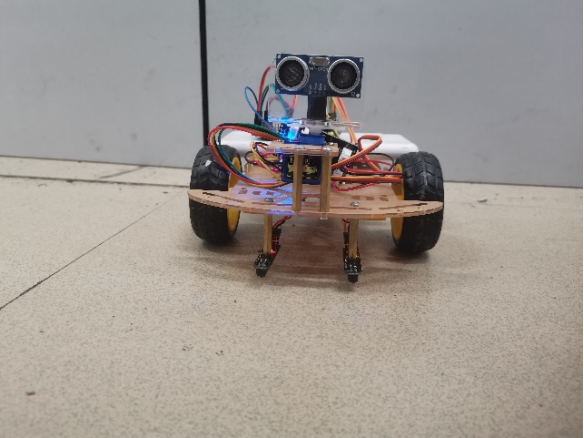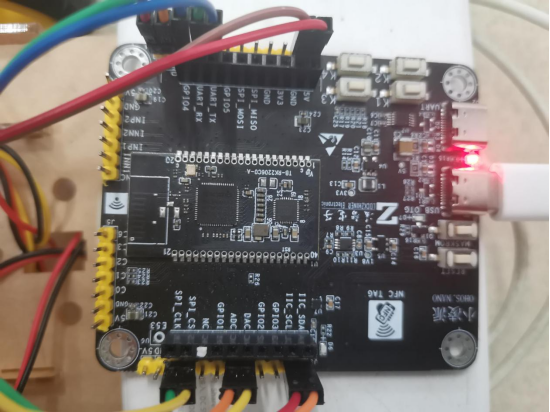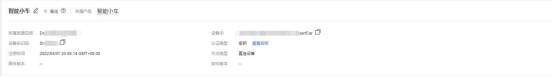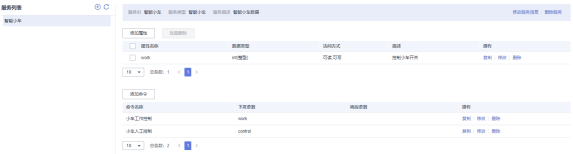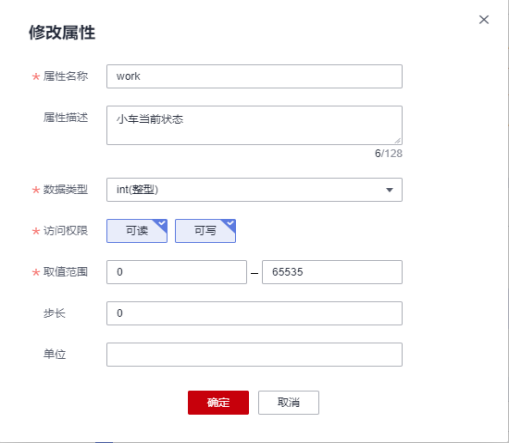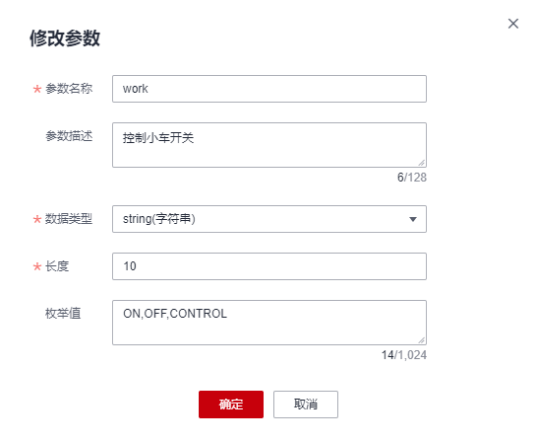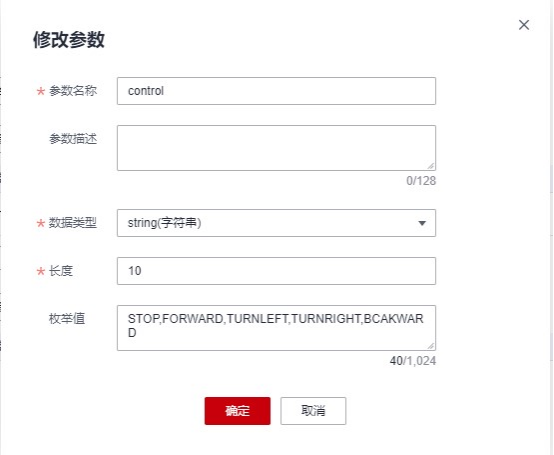1.小车连接华为云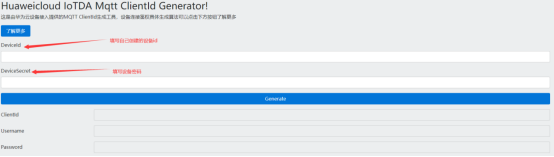``````#define CLIENT_ID

``````

``````#define SSID

``````

2.小车指令接收配置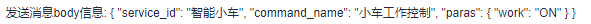``````obj_cmdname = cJSON_GetObjectItem(obj_root, "command_name");
if (obj_cmdname == NULL)
{
goto EXIT;
}
if (0 == strcmp(cJSON_GetStringValue(obj_cmdname), "小车工作控制"))
{
obj_paras = cJSON_GetObjectItem(obj_root, "paras");
if (obj_paras == NULL)
{
goto EXIT;
}
obj_para = cJSON_GetObjectItem(obj_paras, "work");
if (obj_para == NULL)
{
goto EXIT;
}
if (0 == strcmp(cJSON_GetStringValue(obj_para), "ON"))
{
m_app_status.work = 1;
g_car_status = CAR_TRACE_STATUS;
}
else if (0 == strcmp(cJSON_GetStringValue(obj_para), "OFF"))
{
m_app_status.work = 0;
g_car_status = CAR_STOP_STATUS;
}
else if (0 == strcmp(cJSON_GetStringValue(obj_para), "CONTROL"))
{
m_app_status.work = 2;
g_car_status = CAR_MANUAL_OPERATION_STATUS;
}
cmdret = 0;
}
else if (0 == strcmp(cJSON_GetStringValue(obj_cmdname), "小车人工控制"))
{
obj_paras = cJSON_GetObjectItem(obj_root, "paras");
if (obj_paras == NULL)
{
goto EXIT;
}
obj_para = cJSON_GetObjectItem(obj_paras, "control");
if (obj_para == NULL)
{
goto EXIT;
}
if (0 == strcmp(cJSON_GetStringValue(obj_para), "STOP"))
{
g_car_control = CAR_STOP;
}
else if (0 == strcmp(cJSON_GetStringValue(obj_para), "FORWARD"))
{
g_car_control = CAR_FORWARD;
}
else if (0 == strcmp(cJSON_GetStringValue(obj_para), "TURNLEFT"))
{
g_car_control = CAR_TURNLEFT;
}
else if (0 == strcmp(cJSON_GetStringValue(obj_para), "TURNRIGHT"))
{
g_car_control = CAR_TURNRIGHT;
}
else if (0 == strcmp(cJSON_GetStringValue(obj_para), "BCAKWARD"))
{
g_car_control = CAR_BCAKWARD;
}
cmdret = 0;
}

``````

3.小车数据发送配置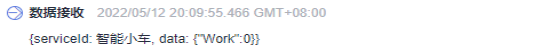`````` oc_mqtt_profile_service_t service;
oc_mqtt_profile_kv_t work;

service.event_time = NULL;
service.service_id = "智能小车";
service.service_property = &work;
service.nxt = NULL;

work.key = "Work";
work.value = &report->work;
work.type = EN_OC_MQTT_PROFILE_VALUE_INT;
work.nxt = NULL;

``````

``````现在通过华为云向小车下发工作命令。从视频中可以看到在小车的前方和左边都有障碍物。启动小车后小车会先直行，通过超声波传感器获取前方的距离，当距离小于20cm时，小车通过超声波传感器测量左右两边的距离，由于右边的距离比左边大，小车会向右转。从视频中可以看到，小车按照我们预想的方向行动，同时我们可以看到华为云接收到的消息为data:{“work”:1}，说明小车现在正在工作。
``````

``````if (distance < DISTANCE_BETWEEN_CAR_AND_OBSTACLE)
{
car_stop();
LOS_Msleep(500);
car_backward();
printf("This is backward\r\n");
LOS_Msleep(500);
car_stop();
unsigned int ret = engine_go_where();
printf("ret is %d\r\n", ret);
if (ret == CAR_TURN_LEFT)
{
car_left();
LOS_Msleep(800);
}
else if (ret == CAR_TURN_RIGHT)
{
car_right();
LOS_Msleep(800);
}
car_stop();
}

``````

2帖子
视频
声望
粉丝
社区精华内容